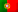Home > Technology > Computer Science > Programming > C++ > Class template in C++

# Class template in C++

The template declaration is C++ allows us to define generic classes and functions. In the case of a class template, we can define classes where we have properties that can assume any types of data or objects. These classes have a special behaviour and the code will only be generated with the compiler founds their usage. Note that, if we use some different types, the compiler will generate code for many classes as the types that we use, generating each one according to each data type.

Let’s consider the following example:

We want to create a class that receives a pair of values from any type (int, float, string, …) and be able to return which one is the greater value (max) and which one is the lesser value (min).

Let’s see how we can define this class:

```template <class T>
class Pair {
T val1;
T val2;
public:
Pair(T v1, T v2) : val1(v1), val2(v2) {}
T max() { return val1 > val2 ? val1 : val2; }
T min() { return val1 < val2 ? val1 : val2; }
};
```

In line 1, we use the declaration “template <class T>” to tell the compiler we have a class template that may receive a generic typeT“. In lines 3 and 4, we define de properties “val1” and “val2” as of that generic type “T”. In the constructor, in line 6, we tell that the parameters are also of that generic type “T”, and the functions max() and min() as well.

If we have two integer variables, i1 and i2, we can use this class as follows:

```    Pair<int> pInt(i1, i2);
cout << "The greater integer is: " << pInt.max() << endl;
cout << "The lesser integer is: " << pInt.min() << endl;
```

Notice the way how we declare “pInt” as being an instance of “Pair<int>”. At this moment, the compiler will generate the code for the class “Pair” where the generic type “T” will be replaced by the type “int”. It is like if we have defined the following class:

```class Pair {
int val1;
int val2;
public:
Par(int v1, int v2) : val1(v1), val2(v2) {}
int max() { return val1 > val2 ? val1 : val2; }
int min() { return val1 < val2 ? val1 : val2; }
};
```

The same would happen if we had used any other type! Giving one more example, imagine that we declared a variable as being an instance of “Pair<string>”. The generated class could be as follows:

```class Pair {
string val1;
string val2;
public:
Par(string v1, string v2) : val1(v1), val2(v2) {}
string max() { return val1 > val2 ? val1 : val2; }
string min() { return val1 < val2 ? val1 : val2; }
};
```

Notice that, all this code is generated at compile time and these two last examples are just that – examples – and they illustrate the equivalent classes in case we didn’t use templates! We don’t see this code being generated.

The following complete program will use three different types for the class template “Pair<T>”:

```#include <iostream>
#include <stdlib.h>
#include <string>

using namespace std;

template <class T>
class Pair {
T val1;
T val2;
public:
Pair(T v1, T v2) : val1(v1), val2(v2) {}
T max() { return val1 > val2 ? val1 : val2; }
T min() { return val1 < val2 ? val1 : val2; }
};

int main() {
int i1, i2;
float f1, f2;
string s1, s2;
cout << "Insert 2 integers:" << endl;
cin >> i1;
cin >> i2;
Pair<int> pInt(i1, i2);
cout << "The greater integer is: " << pInt.max() << endl;
cout << "The lesser integer is: " << pInt.min() << endl;
cout << "Insert 2 floats:" << endl;
cin >> f1;
cin >> f2;
Pair<float> pFloat(f1, f2);
cout << "The greater float is: " << pFloat.max() << endl;
cout << "The lesser float is: " << pFloat.min() << endl;
cout << "Introduza 2 strings:" << endl;
cin >> s1;
cin >> s2;
Pair<string> pStr(s1, s2);
cout << "The greater string is: " << pStr.max() << endl;
cout << "The lesser string is: " << pStr.min() << endl;
}
```

Example of the result of the program’s execution:

Insert 2 integers:
40
30
The greater integer is: 40
The lesser integer is: 30
Insert 2 floats:
6.5
3.4
The greater float is: 6.5
The lesser float is: 3.4
Introduza 2 strings:
abc
def
The greater string is: def
The lesser string is: abc

Important note: When we declare our classes in separated files, we usually write the class’ definition code in a header file “.h” and the implementation code in a “.cpp” file. When we use class templates, we can’t do this separation because the compiler needs to generate everything together. Otherwise, during the linkage phase, an error will be thrown because the linker won’t be able to find the implementation generated by the compiler! In this case, just write everything together in a “.h” file.

To learn about function templates, read the following article: “Function templates in C++

Follow us on the social networks and stay tuned with news from our multi-thematic blog – Out4Mind!